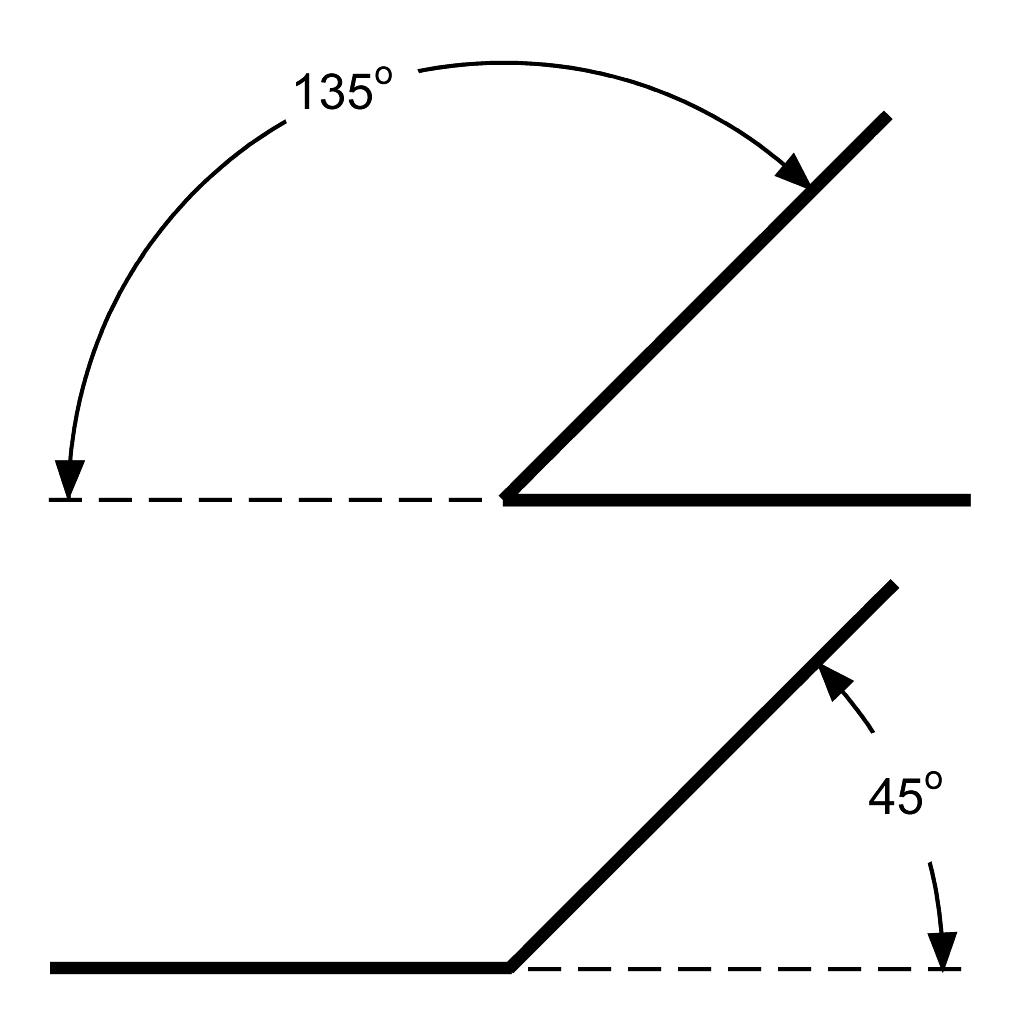The Octagonal SystemOct Angles make Octagons, or a 45o transition.
Octagonal complements are a 135o transition.

• 12 sizes: 1" to 12" in 1 inch increments.
• Labeled: size is stamped into the metal for easy identification.
• Oversized: sized up 1/8" to include a layer of fiber.
• Stainless steel: long life, stable in the kiln.
• Light guage material: easily make other shapes or true up old ones.

Oct (45o) Equal Leg Angles

 SC_A45_1 #1 equal leg angle \$ 2.00
 SC_A45_2 #2 equal leg angle \$ 3.20
 SC_A45_3 #3 equal leg angle \$ 4.40
 SC_A45_4 #4 equal leg angle \$ 5.60
 SC_A45_5 #5 equal leg angle \$ 6.80
 SC_A45_6 #6 equal leg angle \$ 8.00
 SC_A45_7 #7 equal leg angle \$ 9.20
 SC_A45_8 #8 equal leg angle \$ 10.40
 SC_A45_9 #9 equal leg angle \$ 11.60
 SC_A45_10 #10 equal leg angle \$ 12.80
 SC_A45_11 #11 equal leg angle \$ 14.00
 SC_A45_12 #12 equal leg angle \$ 15.20

Oct Complements (135o) Equal Leg Angles

 SC_A135_1 #1 equal leg angle \$ 2.00
 SC_A135_2 #2 equal leg angle \$ 3.20
 SC_A135_3 #3 equal leg angle \$ 4.40
 SC_A135_4 #4 equal leg angle \$ 5.60
 SC_A135_5 #5 equal leg angle \$ 6.80
 SC_A135_6 #6 equal leg angle \$ 8.00
 SC_A135_7 #7 equal leg angle \$ 9.20
 SC_A135_8 #8 equal leg angle \$ 10.40
 SC_A135_9 #9 equal leg angle \$ 11.60
 SC_A135_10 #10 equal leg angle \$ 12.80
 SC_A135_11 #11 equal leg angle \$ 14.00
 SC_A135_12 #12 equal leg angle \$ 15.20